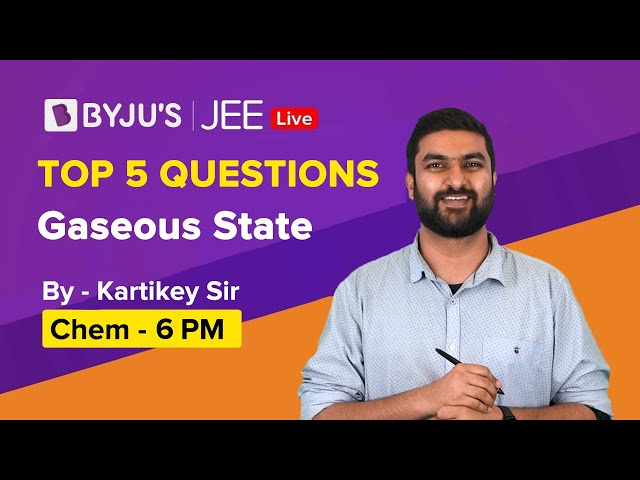# Important Gaseous State Formulas For JEE Main and Advanced

Here is the list of all Gaseous State formulas. The list is very helpful in helping students revise all the important points quickly before the JEE Main or Advanced Exam.

## Gaseous State Formulas

1. Temperature Scale :

$\frac{C-0}{100-0} = \frac{K-273}{373-273}=\frac{ F-32}{212-32}=\frac{R-R(0)}{R(100) – R(0)}$

Here, R = Temp. on an unknown scale.

1. Boyle’s law and measurement of pressure:

At constant temperature,

$V \alpha \frac{1}{ P }$

$P _{1} V _{1}= P _{2} V _{2}$

1. Charles law:

At constant pressure,

$V \propto T$

OR

$\frac{V_{1}}{T_{1}}=\frac{V_{2}}{T_{2}}$

1. Gay-lussac’s law:

At constant volume,

$\quad P \alpha T$

$\frac{ P _{1}}{ T _{1}}=\frac{ P _{2}}{ T _{2}} \rightarrow temp\: on\: absolute\: scale$

1. Ideal gas Equation:

PV = nRT

$PV =\frac{ w }{ m } RT\: Or\: P =\frac{ d }{ m } RT\: Or\: Pm = dRT$

1. Dalton’s law of partial pressure:

$P _{1}=\frac{ n _{1} RT }{ v }, \quad P _{2}=\frac{ n _{2} RT }{ v }, \quad P _{3}=\frac{ n _{3} RT }{ v }$ and so on.

Total pressure = $P _{1}+ P _{2}+ P _{3}+\ldots \ldots$.

Partial pressure = mole fraction X Total pressure.

1. Amagat’s law of partial volume:

$V = V _{1}+ V _{2}+ V _{3}+\ldots \ldots$.

1. Average molecular mass of gaseous mixture:

$M _{ mx }=\frac{\text { Total mass of mixture }}{\text { Total no. of moles in mixture }} =\frac{ n _{1} M _{1}+ n _{2} M _{2}+ n _{3} M _{3}}{ n _{1}+ n _{2}+ n _{3}}$

1. Graham’s Law:

$Rate\: of\: diffusion\: r \propto \frac{1}{\sqrt{ d }} ; d = density of gas$

$\frac{r_{1}}{r_{2}}=\frac{\sqrt{d_{2}}}{\sqrt{d_{1}}}=\frac{\sqrt{M_{2}}}{\sqrt{M_{1}}}=\sqrt{\frac{V \cdot D_{2}}{V \cdot D_{1}}}$

1. Kinetic Theory of Gases:
• $PV =\frac{1}{3} mN \overline{ U ^{2}} \quad$ Kinetic equation of gases
• Average K.E. for one mole = $N_{A}\left(\frac{1}{2} m \bar{U}^{2}\right)=\frac{3}{2} K N_{A} T=\frac{3}{2} R T$
• Root mean square speed
$U_{ rms }=\sqrt{\frac{3 RT }{ M }}$
molar mass must be in kg/mole.
• Average speed
$U _{ av }= U _{1}+ U _{2}+ U _{3}+\ldots \ldots \ldots \ldots U _{ N }$
$U_{\text {avg. }}=\sqrt{\frac{8 R T}{\pi M}}=\sqrt{\frac{8 K T}{\pi m}}$

K is Boltzmman constant

• Most probable speed
$U_{ MPS }=\sqrt{\frac{2 RT }{ M }}=\sqrt{\frac{2 KT }{ m }}$
1. Van Der wall’s equation:

$\left(P+\frac{a n^{2}}{v^{2}}\right)(v-n b)=n R T$

1. Critical constants:

$V_{c}=3 b$,

$P_{c}=\frac{a}{27 b^{2}}$,

$T _{ c }=\frac{8 a }{27 Rb }$

1. Van Der Waal equation in virial form :

$z=\left(1+\frac{b}{V_{m}}+\frac{b^{2}}{V_{m}^{2}}+\frac{b^{3}}{V_{m}^{3}}+\ldots \ldots \ldots\right)-\frac{a}{V_{m} R T} =1+\frac{1}{V_{m}}\left(b-\frac{a}{R T}\right)+\frac{b^{2}}{V_{m}^{2}}+\frac{b^{3}}{V_{m}^{3}}+\ldots \ldots \ldots \ldots \ldots$

1. Reduced Equation of state:

$\left(P_{r}+\frac{3}{V_{r}^{2}}\right)\left(3 V_{r}-1\right)=8 T_{r}$

Up Next: Important Atomic Structure Formulas For JEE

### Gaseous State Question and SolutionsTest Your Knowledge On Important Gaseous State Formulas For Jee!